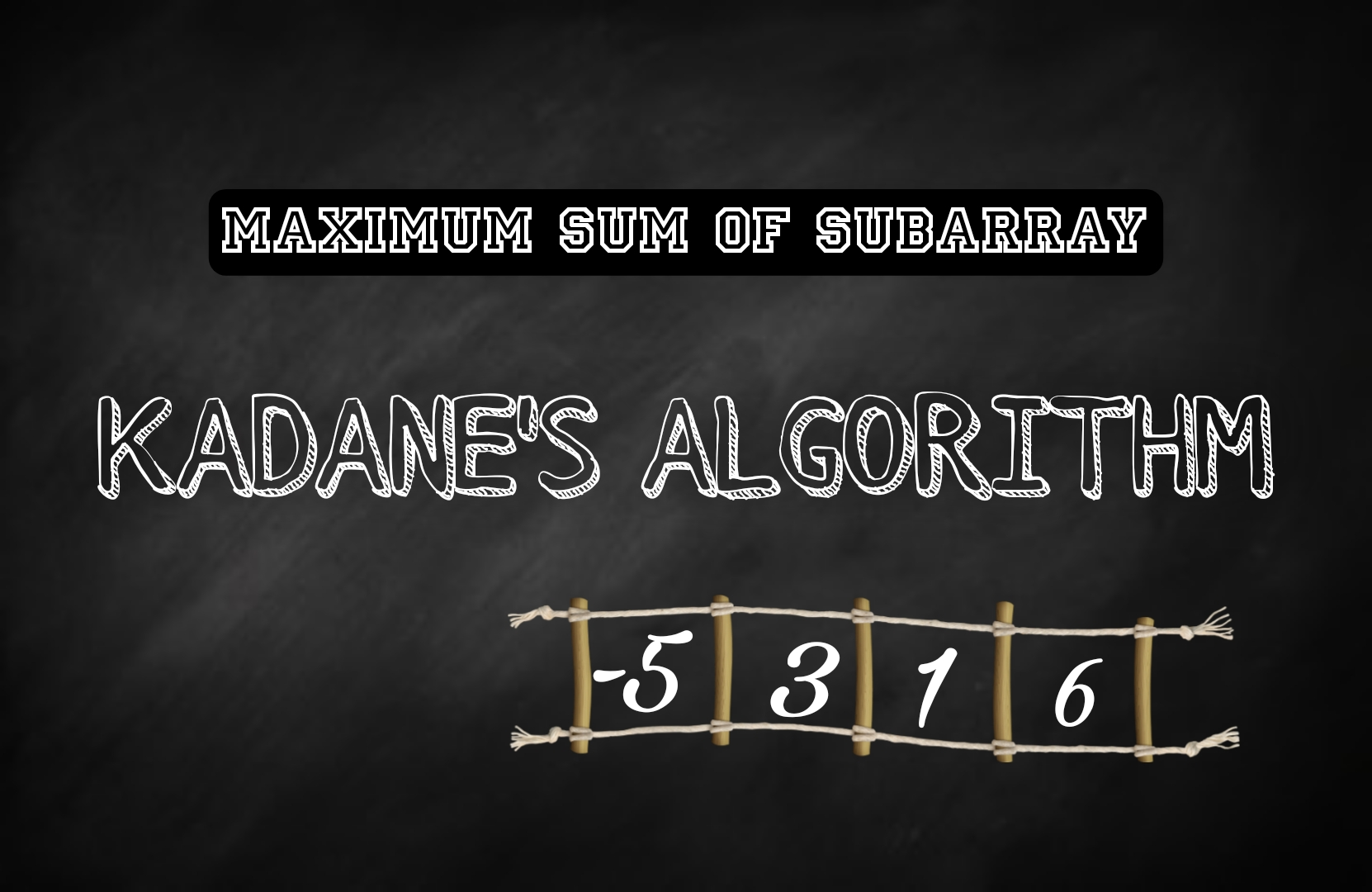# Kadane's algorithm : Maximum sum of Subarray | Easy Solution in C, C++, Java, Python

Kadane's algorithm is a popular algorithm used to find the maximum contiguous subarray sum within a given array of numbers. It efficiently solves the maximum subarray sum problem with a time complexity of O(n), where n is the size of the array.

Note: One of the most important questions for the Interview Process.Kadane's Algorithm

The problem statement is as follows: Given an array of numbers, we want to find the contiguous subarray (subarray with consecutive elements) that has the maximum sum.

Kadane's algorithm works by iteratively scanning through the array and keeping track of the maximum subarray sum encountered so far. It uses two variables: maxSoFar and maxEndingHere.

#### Here's how Kadane's algorithm works:

1. Initialize two variables: maxSoFar and maxEndingHere, both set to the first element of the array.
2. Iterate through the array starting from the second element.
3. For each element, update maxEndingHere by taking the maximum value between the current element and the sum of the current element and maxEndingHere. This step checks if it is more beneficial to start a new subarray from the current element or continue the existing one.
4. Update maxSoFar by taking the maximum value between maxSoFar and maxEndingHere. This step keeps track of the maximum subarray sum encountered so far.
5. Repeat steps 3 and 4 until all elements of the array have been processed.
6. At the end of the iteration, maxSoFar will hold the maximum subarray sum.

### JAVA Solution:

public static int maxSubarraySum(int[] arr) {
int maxSoFar = arr;
int maxEndingHere = arr;

for (int i = 1; i < arr.length; i++) {
maxEndingHere = Math.max(arr[i], maxEndingHere + arr[i]);
maxSoFar = Math.max(maxSoFar, maxEndingHere);
}

return maxSoFar;
}

public static void main(String[] args) {
int[] arr = {-2, 1, -3, 4, -1, 2, 1, -5, 4};
int result = maxSubarraySum(arr);
System.out.println(result); // Output: 6
}
}

### C Solution :

#include <stdio.h>

int maxSubarraySum(int arr[], int size) {
int maxSoFar = arr;
int maxEndingHere = arr;

for (int i = 1; i < size; i++) {
maxEndingHere = (arr[i] > maxEndingHere + arr[i]) ? arr[i] : maxEndingHere + arr[i];
maxSoFar = (maxSoFar > maxEndingHere) ? maxSoFar : maxEndingHere;
}

return maxSoFar;
}

int main() {
int arr[] = {-2, 1, -3, 4, -1, 2, 1, -5, 4};
int size = sizeof(arr) / sizeof(arr);
int result = maxSubarraySum(arr, size);
printf("%d\n", result); // Output: 6

return 0;
}

### C++ Solution :

#include <iostream>
#include <climits>

int maxSubarraySum(int arr[], int size) {
int maxSoFar = arr;
int maxEndingHere = arr;

for (int i = 1; i < size; i++) {
maxEndingHere = std::max(arr[i], maxEndingHere + arr[i]);
maxSoFar = std::max(maxSoFar, maxEndingHere);
}

return maxSoFar;
}

int main() {
int arr[] = {-2, 1, -3, 4, -1, 2, 1, -5, 4};
int size = sizeof(arr) / sizeof(arr);
int result = maxSubarraySum(arr, size);
std::cout << result << std::endl; // Output: 6

return 0;
}

### Python Solution:

def max_subarray_sum(arr):
max_so_far = arr
max_ending_here = arr

for i in range(1, len(arr)):
max_ending_here = max(arr[i], max_ending_here + arr[i])
max_so_far = max(max_so_far, max_ending_here)

return max_so_far

arr = [-2, 1, -3, 4, -1, 2, 1, -5, 4]
result = max_subarray_sum(arr)
print(result)  # Output: 6

Time Complexity:

The time complexity of Kadane's algorithm is O(n), where n is the size of the input array. This is because the algorithm iterates through each element of the array once, performing a constant amount of work for each element.

Space Complexity:

The space complexity of Kadane's algorithm is O(1), meaning it requires constant extra space. The algorithm only uses a few variables to keep track of the maximum subarray sum (maxSoFar), the current subarray sum (maxEndingHere), and a loop counter (i). These variables require a constant amount of space, regardless of the size of the input array.

Overall, Kadane's algorithm is a very efficient algorithm for finding the maximum subarray sum, with linear time complexity and constant space complexity.